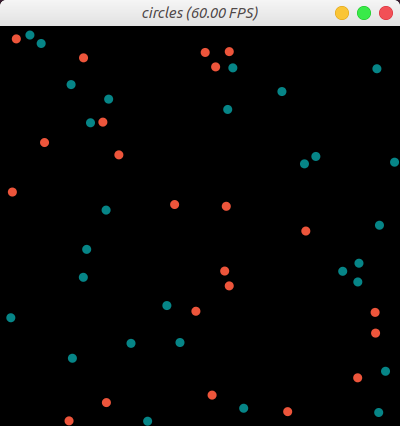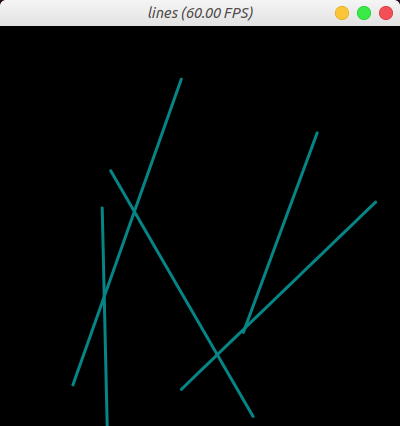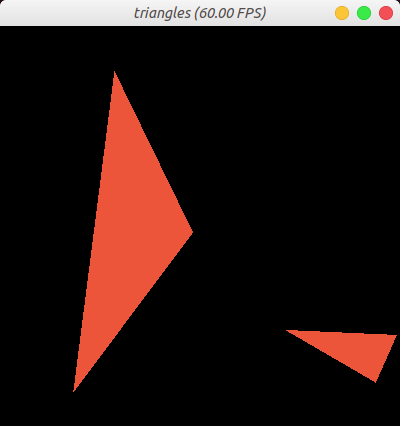Version: v1.1.3

# GUI 系统

Taichi 有一个内置的 GUI 系统，用于对 Taichi fields 或 NumPy ndarray 等数据容器内的数据行进视觉模拟 Taichi GUI 同时对基本几何体的绘制提供了简单的支持。

## 创建并显示窗口

``gui = ti.GUI('Hello World!', (640, 360))while gui.running:    gui.show()``

## 关闭窗口

``gui = ti.GUI('Window Title', (640, 360))while gui.running:    if some_events_happend:        gui.running = False    gui.show()``

## 显示一个 field 或 ndarray

``image = ti.Vector.field(3, ti.f32, shape=(640, 480))while gui.running:    gui.set_image(image)    gui.show()``

### 零复制帧缓存

`gui.et_image()` 方法调用的每个循环内， GUI 系统都会将图像数据转换为可显示的格式，并将结果复制到窗口缓冲区。 当窗口大小较大时，这会造成巨大的超负荷，使得很难实现高 FPS (每秒帧率)。

``gui = ti.GUI(res, title, fast_gui=True)``

• 标量 field `ti.field(dtype, shape)`
• 向量 field `ti.field(3, dtype, shape)` ，兼容 RGB 格式。
• 向量 field `ti.field(4, dtype, shape)` ，兼容 RGBA 格式。

## 在窗口上绘画

Taichi 的 GUI 系统支持绘制简单的几何形状，如线、三角形、长方形、圆圈和文字等。

• `(0.0, 0.0)`: 窗口左下角。
• `(1.0, 1.0)`: 窗口右上角。

``import numpy as nppos = np.random.random((50, 2))# Create an array of 50 integer elements whose values are randomly 0, 1, 2# 0 corresponds to 0x068587# 1 corresponds to 0xED553B# 2 corresponds to 0xEEEEF0indices = np.random.randint(0, 2, size=(50,))gui = ti.GUI("circles", res=(400, 400))while gui.running:    gui.circles(pos, radius=5, palette=[0x068587, 0xED553B, 0xEEEEF0], palette_indices=indices)    gui.show()````import numpy as npX = np.random.random((5, 2))Y = np.random.random((5, 2))gui = ti.GUI("lines", res=(400, 400))while gui.running:    gui.lines(begin=X, end=Y, radius=2, color=0x068587)    gui.show()````import numpy as npX = np.random.random((2, 2))Y = np.random.random((2, 2))Z = np.random.random((2, 2))gui = ti.GUI("triangles", res=(400, 400))while gui.running:    gui.triangles(a=X, b=Y, c=Z, color=0xED553B)    gui.show()``## 事件处理

Taichi的图形界面系统也提供了一套方法用于鼠标和键盘的控制。 输入事件分为三类：

``ti.GUI.RELEASE  # key up or mouse button upti.GUI.PRESS    # key down or mouse button downti.GUI.MOTION   # mouse motion or mouse wheel``

``# for ti.GUI.PRESS and ti.GUI.RELEASE event:ti.GUI.ESCAPE  # Escti.GUI.SHIFT   # Shiftti.GUI.LEFT    # Left Arrow'a'            # we use lowercase for alphabet'b'...ti.GUI.LMB     # Left Mouse Buttonti.GUI.RMB     # Right Mouse Button# for ti.GUI.MOTION event:ti.GUI.MOVE    # Mouse Movedti.GUI.WHEEL   # Mouse Wheel Scrolling``

``# if ESC pressed or released:gui.get_event(ti.GUI.ESCAPE)# if any key is pressed:gui.get_event(ti.GUI.PRESS)# if ESC is pressed or SPACE is released:gui.get_event((ti.GUI.PRESS, ti.GUI.ESCAPE), (ti.GUI.RELEASE, ti.GUI.SPACE))``

`gui.get_event()` 将一个事件从队列中抛出并保存到 `gui.event`。 For example:

``if gui.get_event():    print('Got event, key =', gui.event.key)``

``gui = ti.GUI('Title', (640, 480))while not gui.get_event(ti.GUI.ESCAPE):    gui.set_image(img)    gui.show()``

`gui.is_pressed()` 检测到按键。 如下代码片段所示，您必须将它与 `gui.get_event()` 一起调用。 否则，按键将不会被更新。 For example:

``while True:    gui.get_event()  # must be called before is_pressed    if gui.is_pressed('a', ti.GUI.LEFT):        print('Go left!')    elif gui.is_pressed('d', ti.GUI.RIGHT):        print('Go right!')``
##### warning

For example:

``while True:    gui.get_event() # must be called before is_pressed    if gui.is_pressed('a', ti.GUI.LEFT):        print('Go left!')    elif gui.is_pressed('d', ti.GUI.RIGHT):        print('Go right!')``

#### 获取光标位置

`gui.get_cursor_pos()` 返回光标在当前窗口的位置。 返回值是范围内 `[0.0, 1.0]` 的一对浮点数。 For example:

``mouse_x, mouse_y = gui.get_cursor_pos()``

## GUI 窗口部件

Taichi 的 GUI 系统也提供了包括 `slider()`, `label()`, 和 `buton()` 在内的窗口部件, 方便您自定义您的控制界面。 请看以下代码片段:

``import taichi as tigui = ti.GUI('GUI widgets')radius = gui.slider('Radius', 1, 50, step=1)xcoor = gui.label('X-coordinate')okay = gui.button('OK')xcoor.value = 0.5radius.value = 10while gui.running:    for e in gui.get_events(gui.PRESS):        if e.key == gui.ESCAPE:            gui.running = False        elif e.key == 'a':            xcoor.value -= 0.05        elif e.key == 'd':            xcoor.value += 0.05        elif e.key == 's':            radius.value -= 1        elif e.key == 'w':            radius.value += 1        elif e.key == okay:            print('OK clicked')    gui.circle((xcoor.value, 0.5), radius=radius.value)    gui.show()``